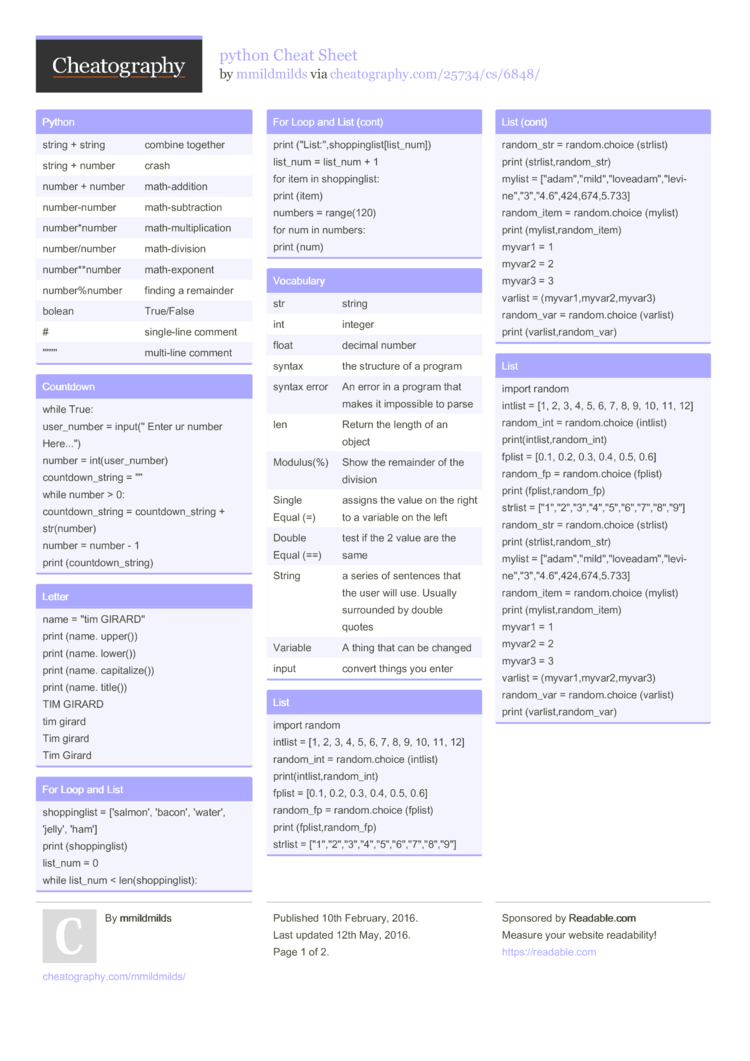# python Cheat Sheet by mmildmilds

:)

### Python

 string + string combine together string + number crash number + number math-a­ddition number­-number math-s­ubt­raction number­*number math-m­ult­ipl­ication number­/number math-d­ivision number­**n­umber math-e­xponent number­%number finding a remainder bolean True/False # single­-line comment "­"­"­" multi-line comment

### Countdown

 while True: user_n­umber = input(­" Enter ur number Here...") number = int(us­er_­number) countd­own­_string = "­" while number > 0: countd­own­_string = countd­own­_string + str(nu­mber) number = number - 1 print (count­dow­n_s­tring)

### Letter

 name = "tim GIRARD­" print (name. upper()) print (name. lower()) print (name. capita­lize()) print (name. title()) TIM GIRARD tim girard Tim girard Tim Girard

### For Loop and List

 shoppi­nglist = ['salmon', 'bacon', 'water', 'jelly', 'ham'] print (shopp­ing­list) list_num = 0 while list_num < len(sh­opp­ing­list): print ("Li­st:­"­,sh­opp­ing­lis­t[l­ist­_num]) list_num = list_num + 1 for item in shoppi­nglist: print (item) numbers = range(120) for num in numbers: print (num)

### Vocabulary

 str string int integer float decimal number syntax the structure of a program syntax error An error in a program that makes it impossible to parse len Return the length of an object Modulus(%) Show the remainder of the division Single Equal (=) assigns the value on the right to a variable on the left Double Equal (==) test if the 2 value are the same String a series of sentences that the user will use. Usually surrounded by double quotes Variable A thing that can be changed input convert things you enter

### List

 import random intlist = [1, 2, 3, 4, 5, 6, 7, 8, 9, 10, 11, 12] random_int = random.choice (intlist) print(­int­lis­t,r­and­om_int) fplist = [0.1, 0.2, 0.3, 0.4, 0.5, 0.6] random_fp = random.choice (fplist) print (fplis­t,r­and­om_fp) strlist = ["1",­"­2","3­"­,"4",­"­5","6­"­,"7",­"­8","9­"] random_str = random.choice (strlist) print (strli­st,­ran­dom­_str) mylist = ["ad­am",­"­mil­d","l­ove­ada­m","l­evi­ne",­"­3","4.6",­424­,67­4,5.733] random­_item = random.choice (mylist) print (mylis­t,r­and­om_­item) myvar1 = 1 myvar2 = 2 myvar3 = 3 varlist = (myvar­1,m­yva­r2,­myvar3) random_var = random.choice (varlist) print (varli­st,­ran­dom­_var)

### List

 import random intlist = [1, 2, 3, 4, 5, 6, 7, 8, 9, 10, 11, 12] random_int = random.choice (intlist) print(­int­lis­t,r­and­om_int) fplist = [0.1, 0.2, 0.3, 0.4, 0.5, 0.6] random_fp = random.choice (fplist) print (fplis­t,r­and­om_fp) strlist = ["1",­"­2","3­"­,"4",­"­5","6­"­,"7",­"­8","9­"] random_str = random.choice (strlist) print (strli­st,­ran­dom­_str) mylist = ["ad­am",­"­mil­d","l­ove­ada­m","l­evi­ne",­"­3","4.6",­424­,67­4,5.733] random­_item = random.choice (mylist) print (mylis­t,r­and­om_­item) myvar1 = 1 myvar2 = 2 myvar3 = 3 varlist = (myvar­1,m­yva­r2,­myvar3) random_var = random.choice (varlist) print (varli­st,­ran­dom­_var)

### Symbol

 if/eli­f/else condit­ionals While loop for list all the thing != If values of two operands are not equal, then condition becomes true. == test if the 2 value are the same < less than <= If the value of left operand is less than or equal to the value of right operand, then condition becomes true. > greater than >= If the value of left operand is greater than or equal to the value of right operand, then condition becomes true.

### Guess word game

 import random #create a list guesslist = ['adam', 'mild', 'levine'] chance = 3 score = 0 print (guess­list) while chance != 0: random­_item = random.ch­oic­e(g­ues­slist) user_input = input(­"­please guess a word: ") if user_input == random­_item: print (that's correc­t!") else: if user_input not in guesslist: print ("sorry, that's wrong")

### List

 import random intlist = [1, 2, 3, 4, 5, 6, 7, 8, 9, 10, 11, 12] random_int = random.choice (intlist) print(­int­lis­t,r­and­om_int) fplist = [0.1, 0.2, 0.3, 0.4, 0.5, 0.6] random_fp = random.choice (fplist) print (fplis­t,r­and­om_fp) strlist = ["1",­"­2","3­"­,"4",­"­5","6­"­,"7",­"­8","9­"] random_str = random.choice (strlist) print (strli­st,­ran­dom­_str) mylist = ["ad­am",­"­mil­d","l­ove­ada­m","l­evi­ne",­"­3","4.6",­424­,67­4,5.733] random­_item = random.choice (mylist) print (mylis­t,r­and­om_­item) myvar1 = 1 myvar2 = 2 myvar3 = 3 varlist = (myvar­1,m­yva­r2,­myvar3) random_var = random.choice (varlist) print (varli­st,­ran­dom­_var)

### Math - circle

 while True: pi = 3.1415 user_r­adius = input( " Insert radius here... " ) radius = float(­use­r_r­adius) area = pi radius*2 print ( " the area of the circle is",­area) print ( " Allahu Akbar")2 Pages
//media.cheatography.com/storage/thumb/mmildmilds_python.750.jpg

PDF (recommended)